# RS Aggarwal Solutions for Class 6 Maths Chapter 7 Decimals

## Class 6 RS Aggarwal Chapter 7 – Decimals

RS Aggarwal Solutions for Class 6 Maths, Chapter 7 are provided here. Students are advised to go through the RS Aggarwal Solutions to prepare for the examination and score high marks. Practising the RS Aggarwal Solutions diligently will help students to gain high marks in the examination. By referring to RS Aggarwal Solutions students get to know about the chapter in detail. Practising textbook questions help the students to boost their self- confidence and to learn the chapters in depth.

Students can refer the RS Aggarwal Solutions for Class 6 Chapter 7 Decimals in pdf format which have been solved by the experts at BYJUS thoroughly. Download PDF of Class 6 Chapter 7 in their respective links.

## Download PDF of RS Aggarwal Solutions for Class 6 Maths Chapter 7 Decimals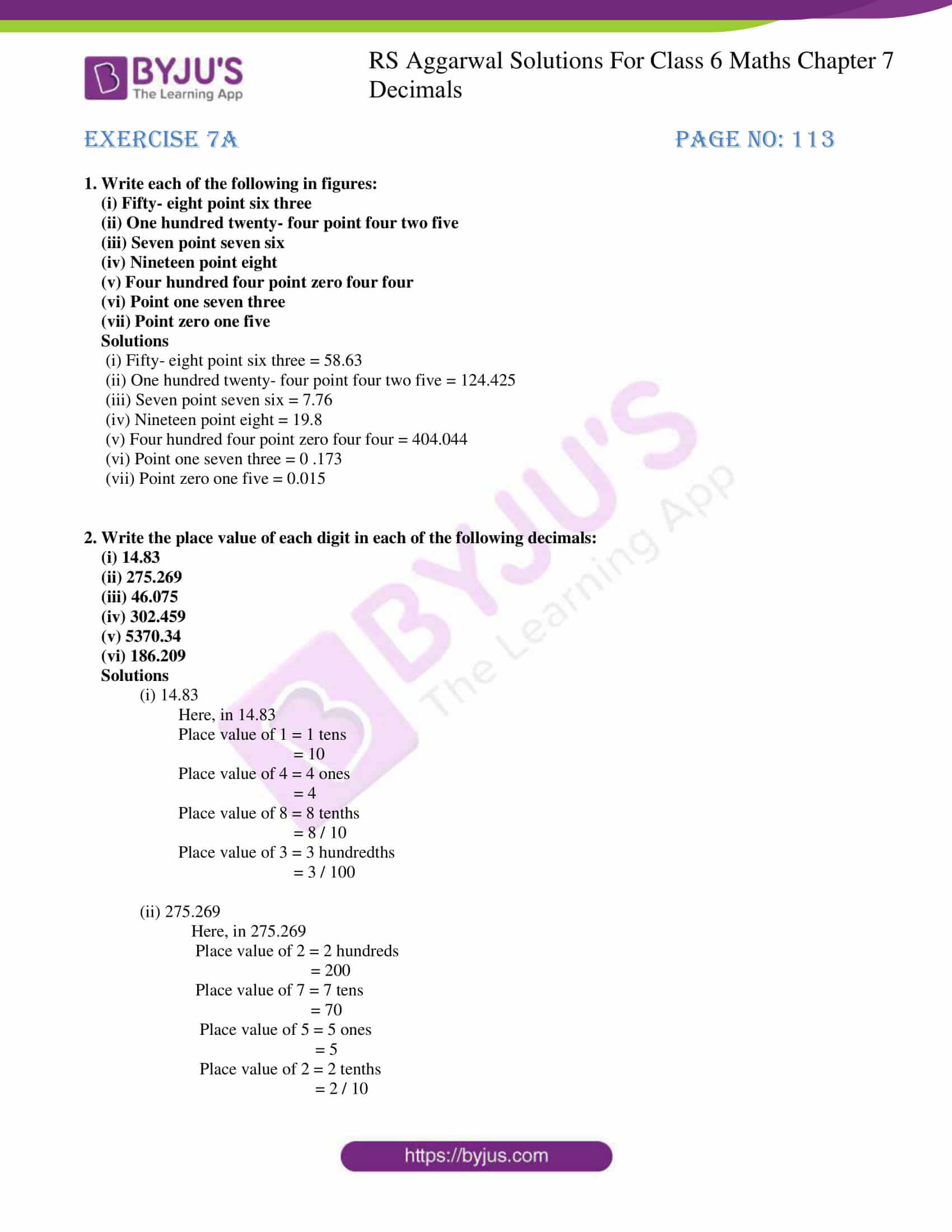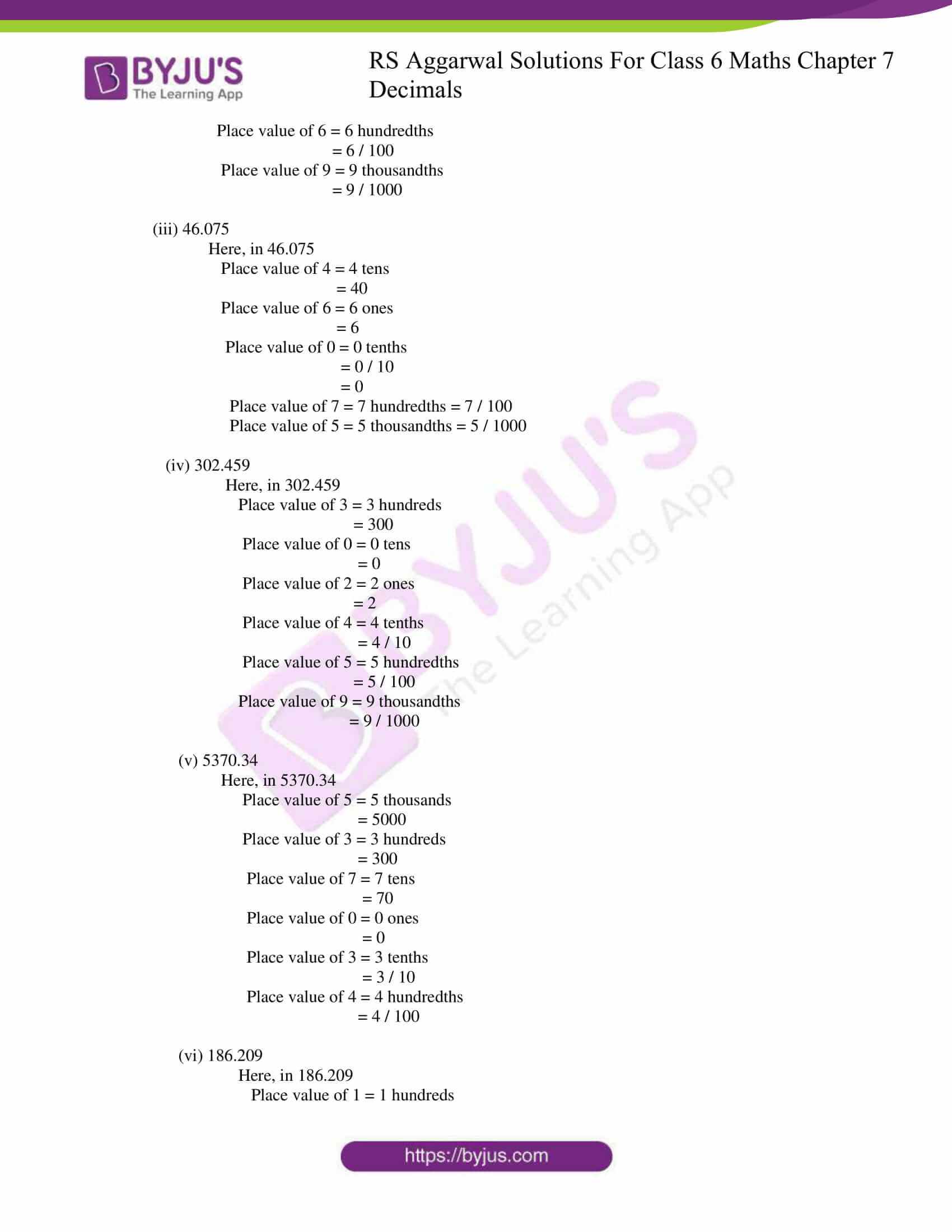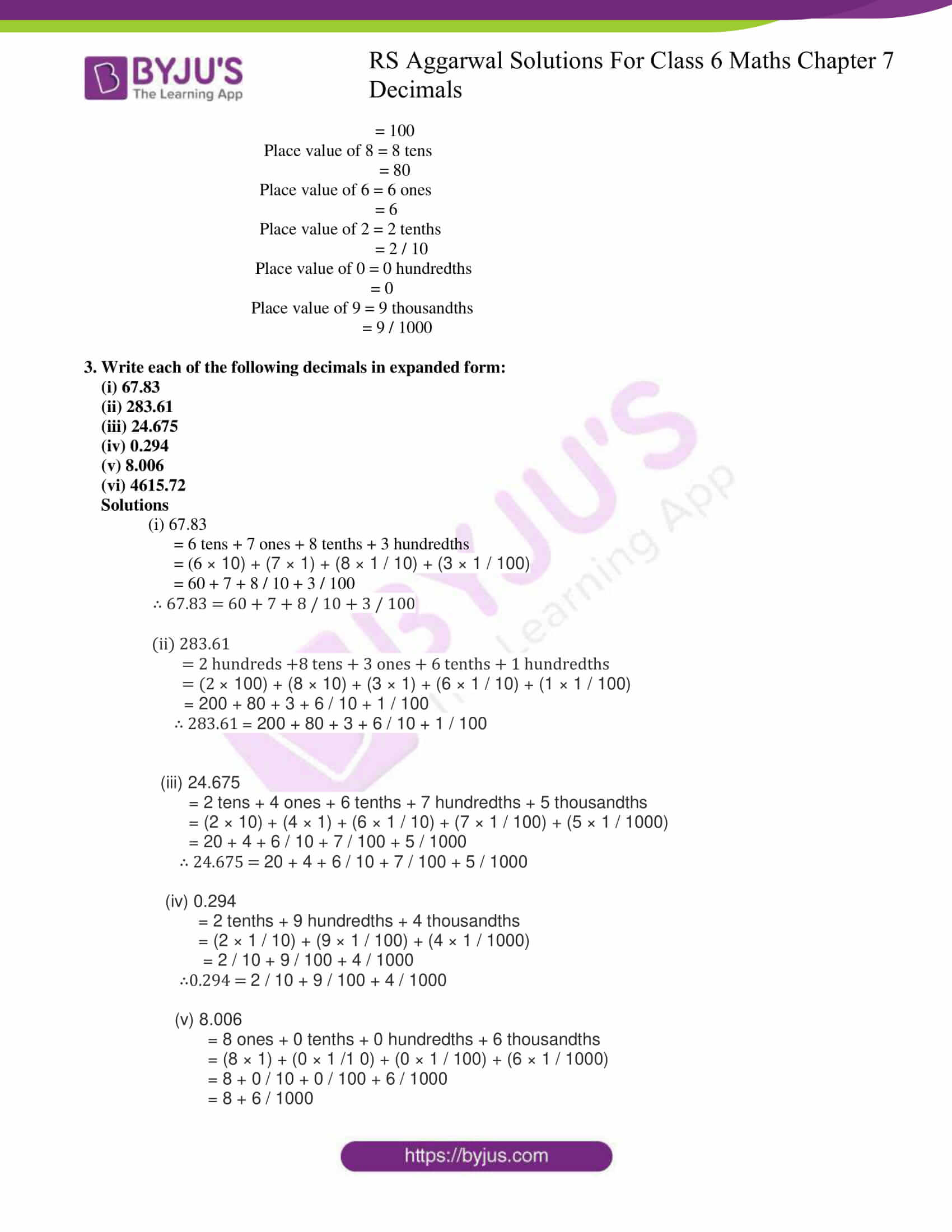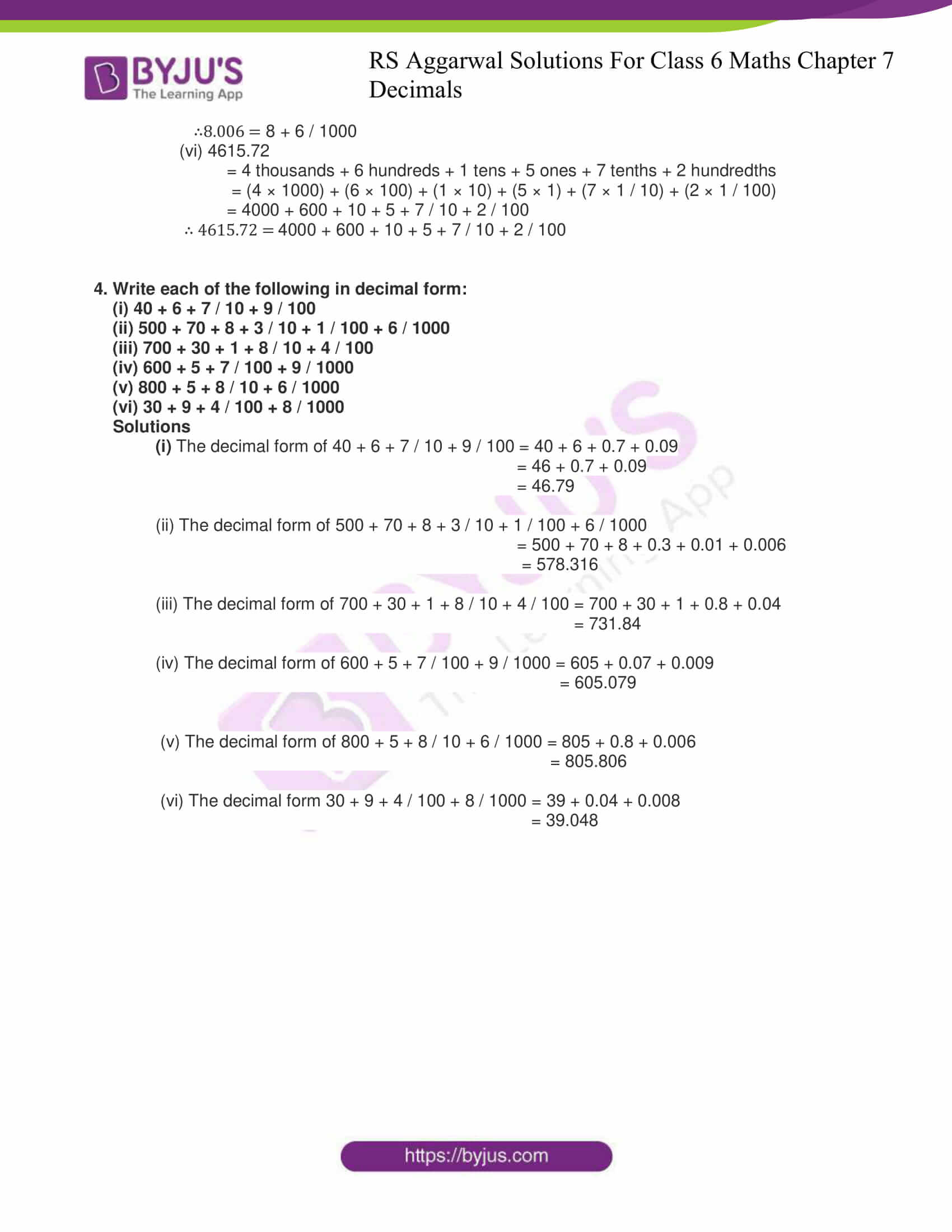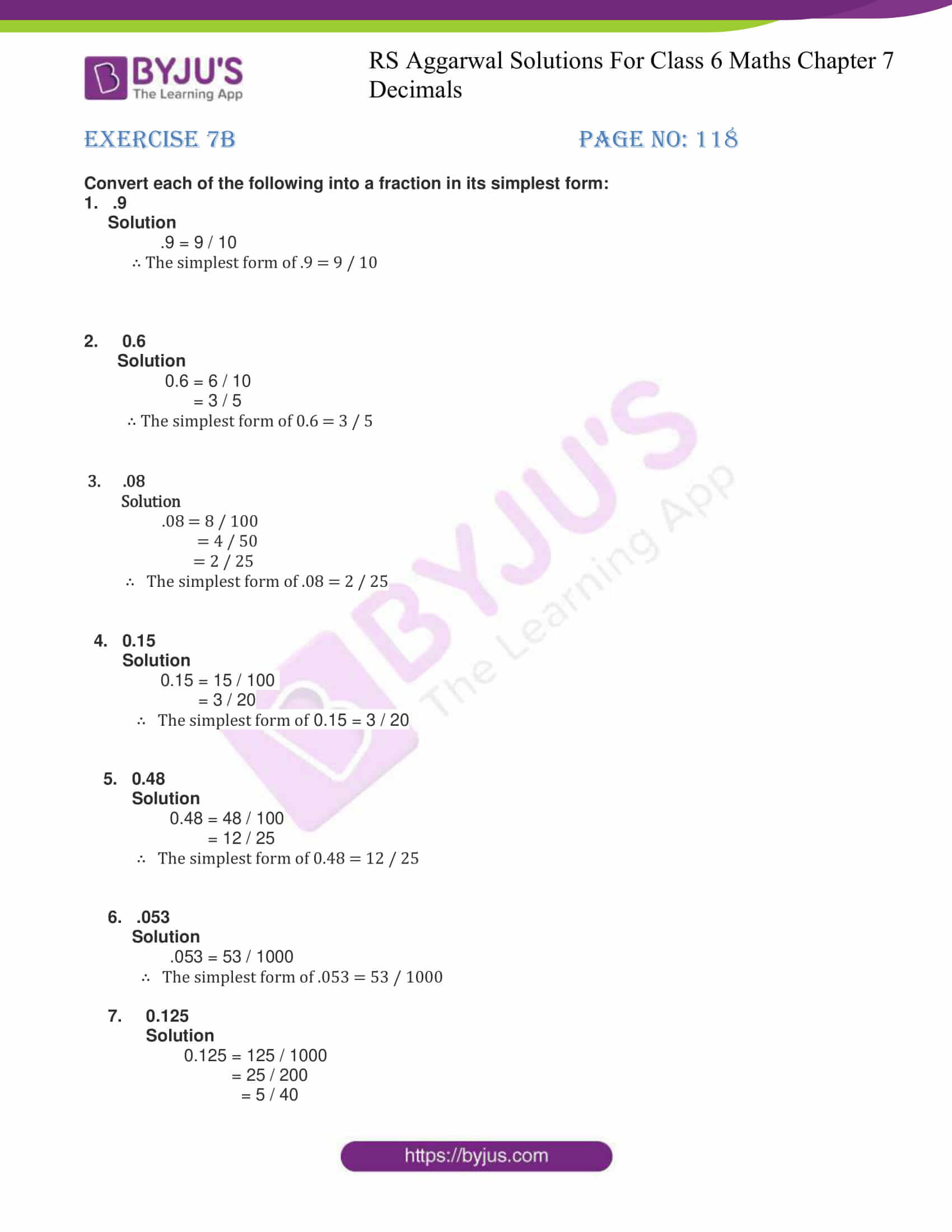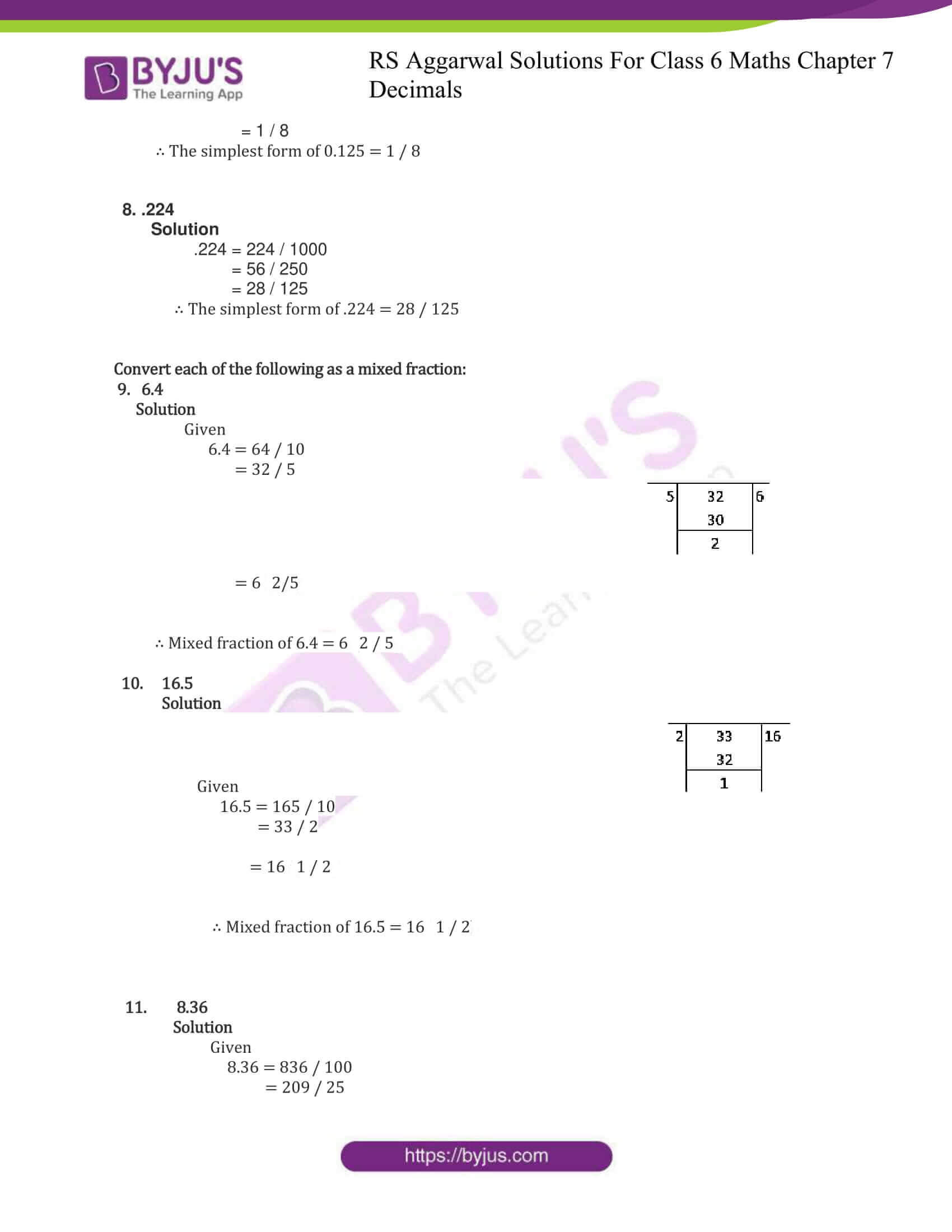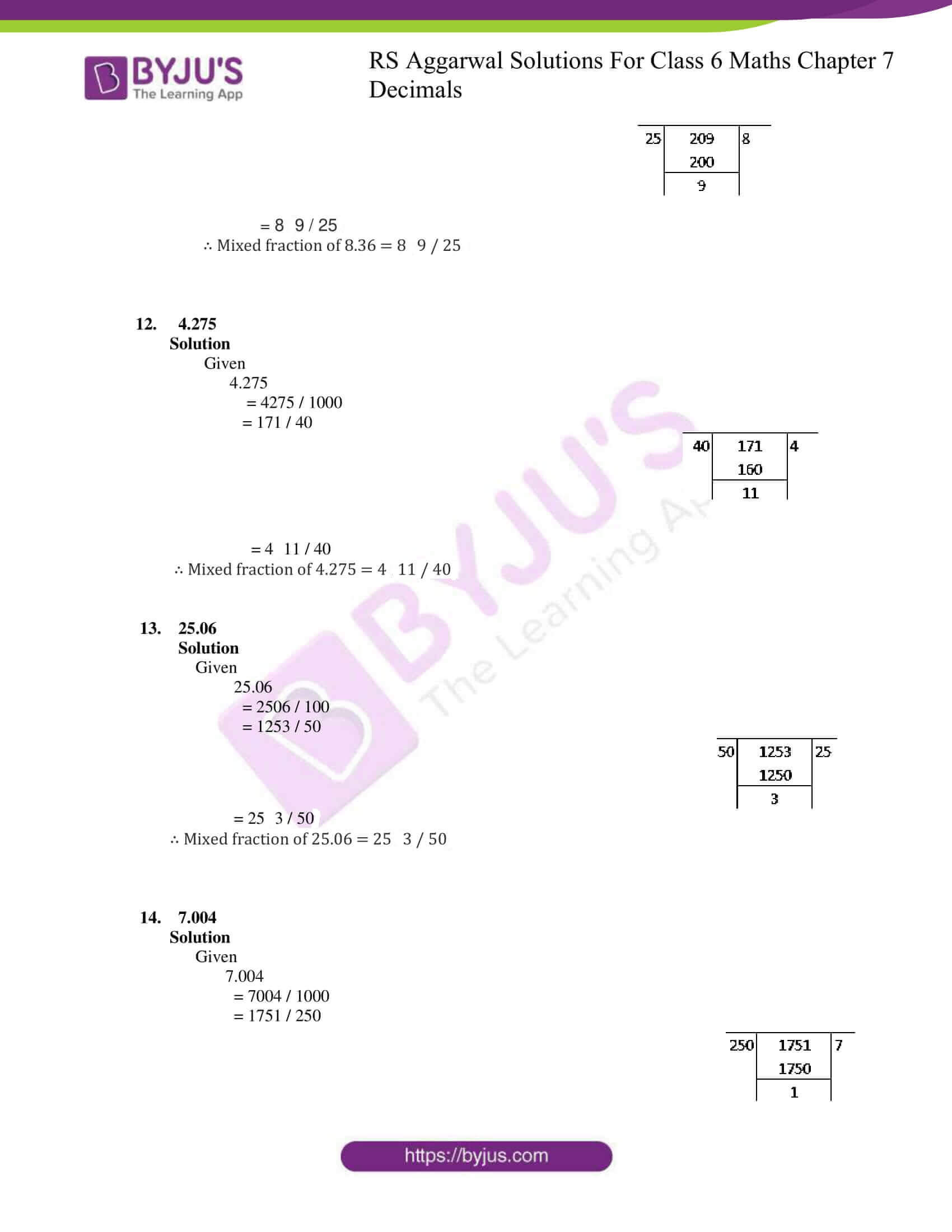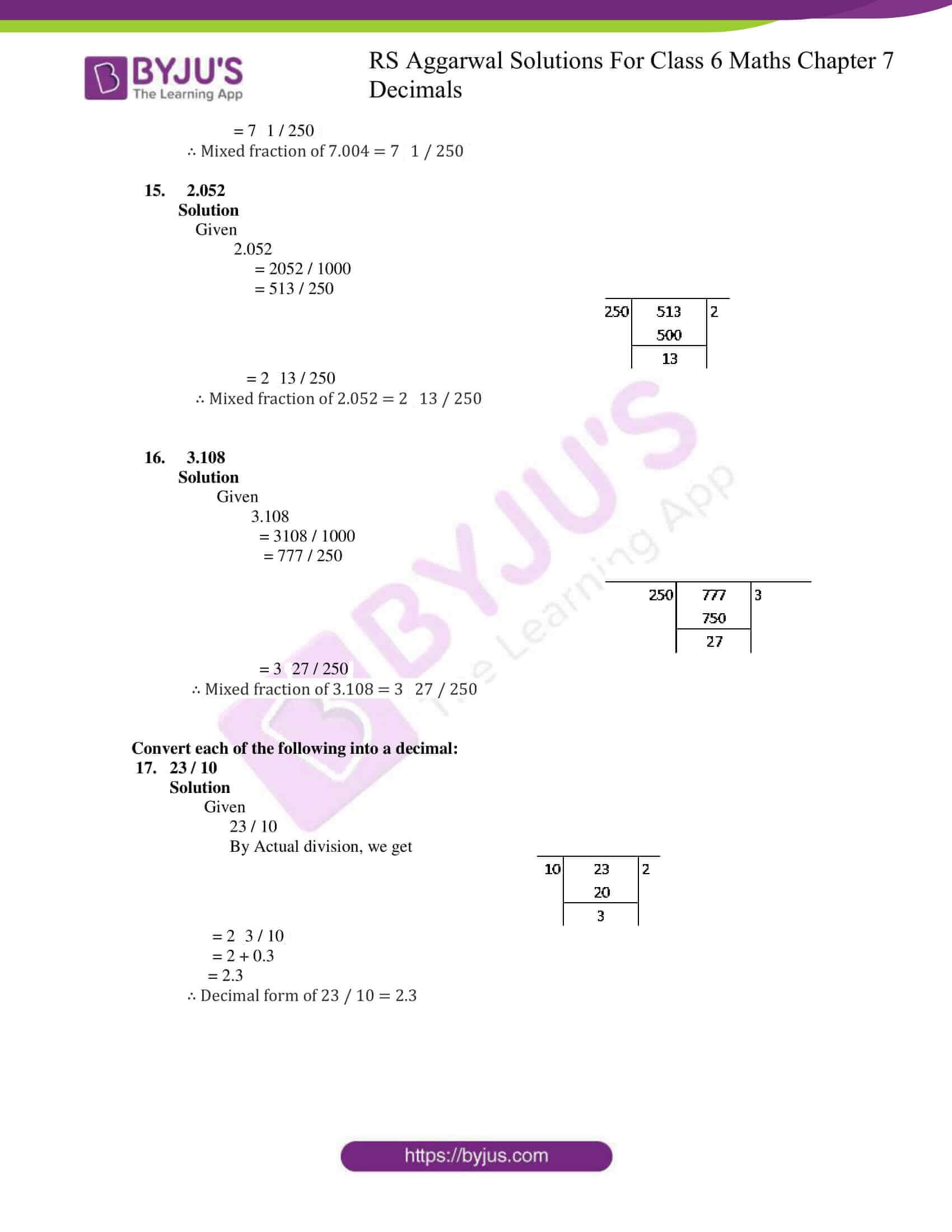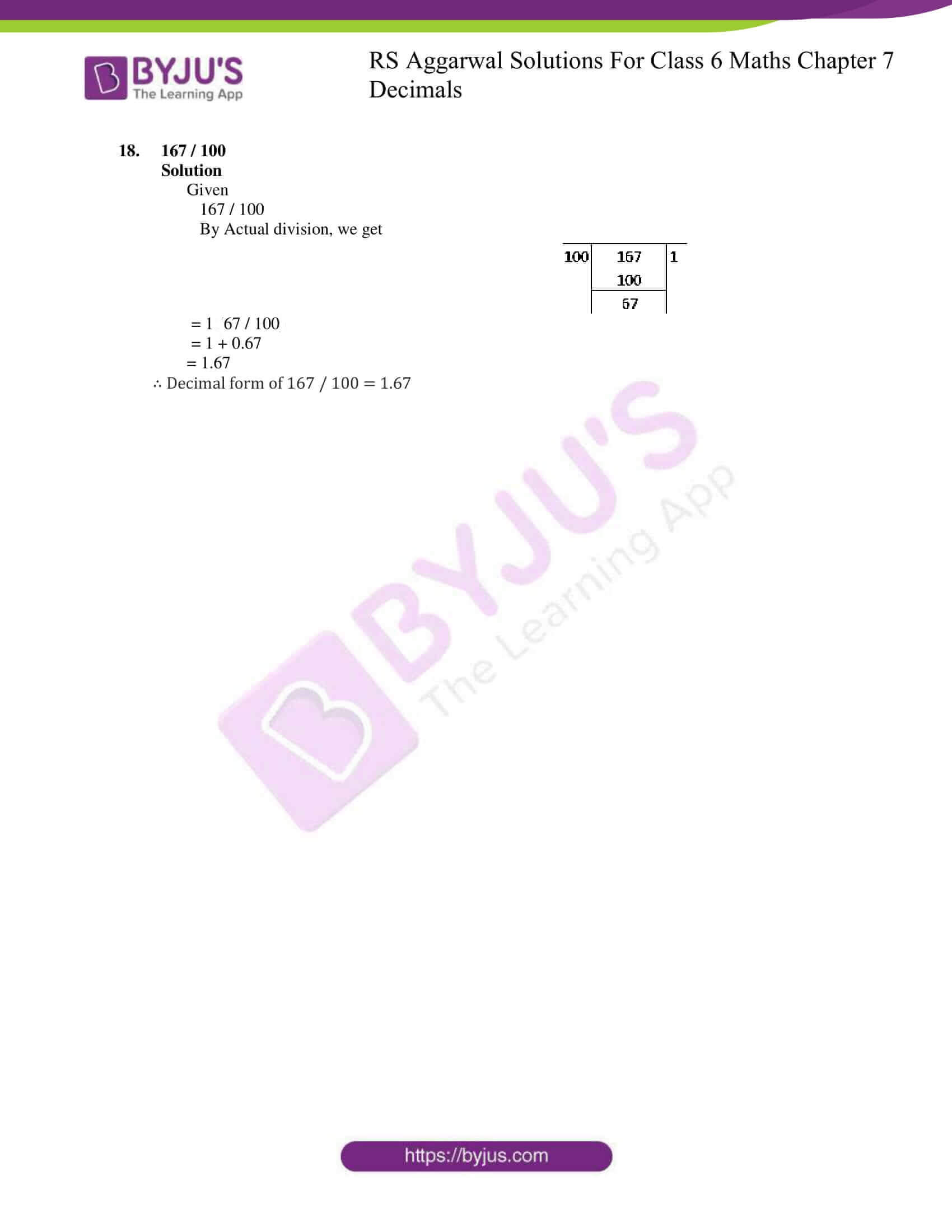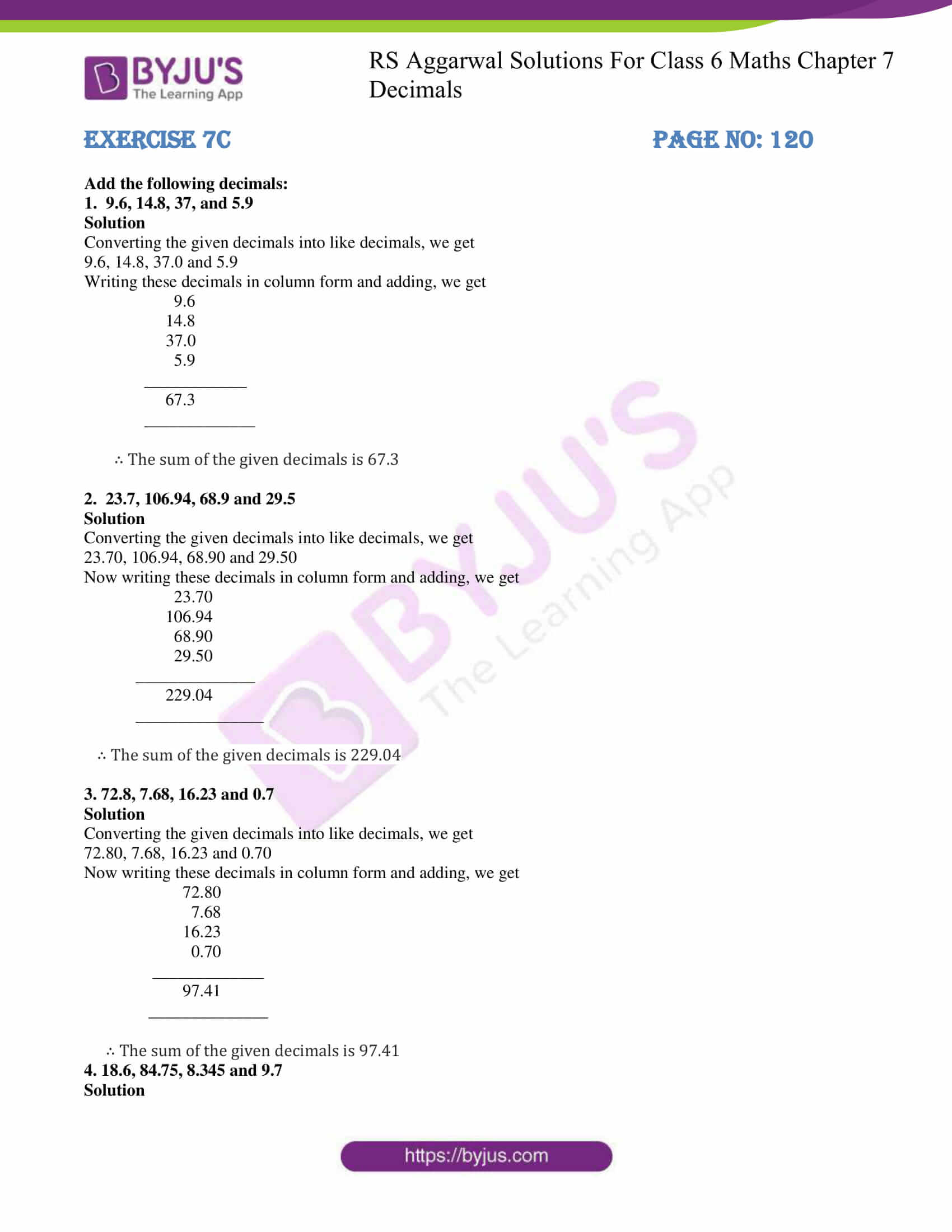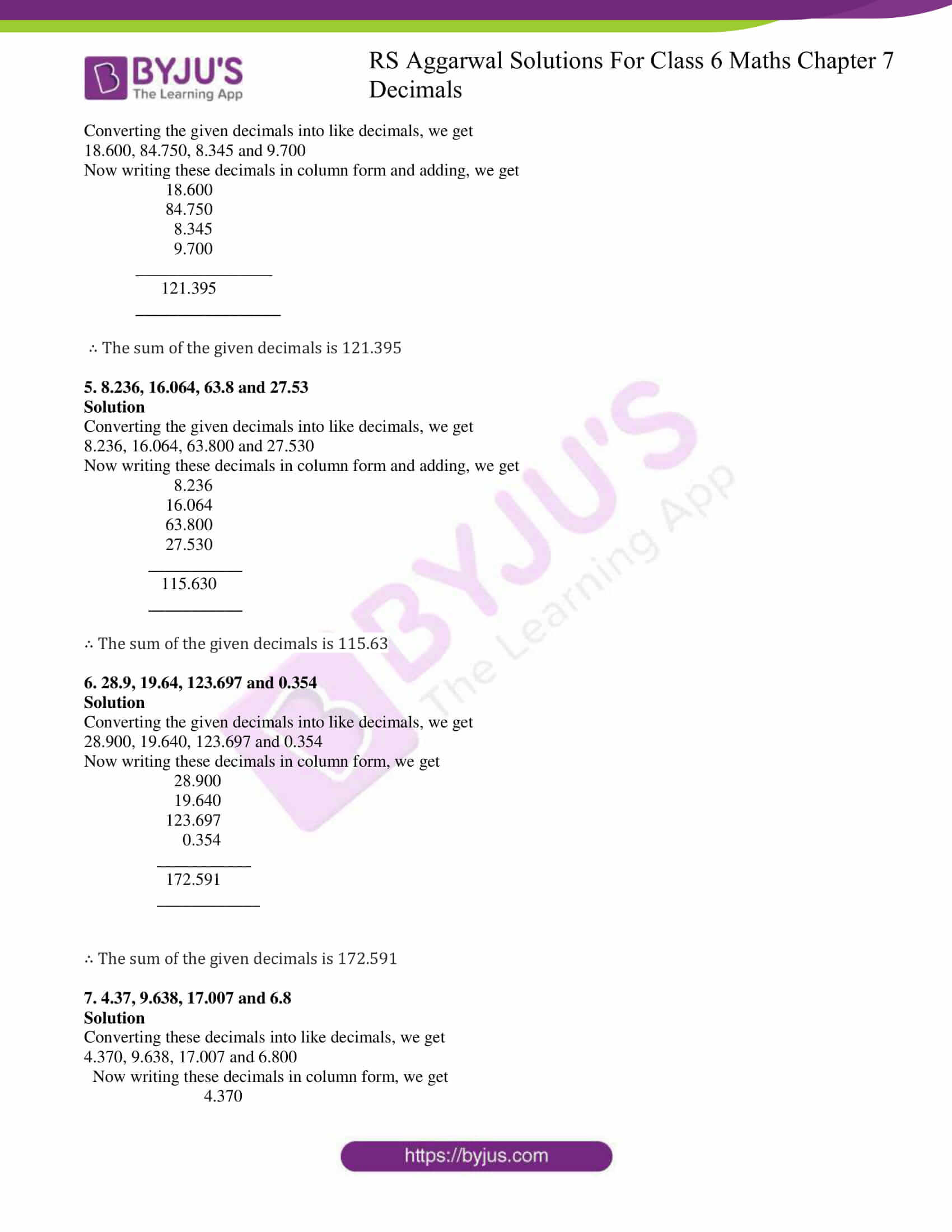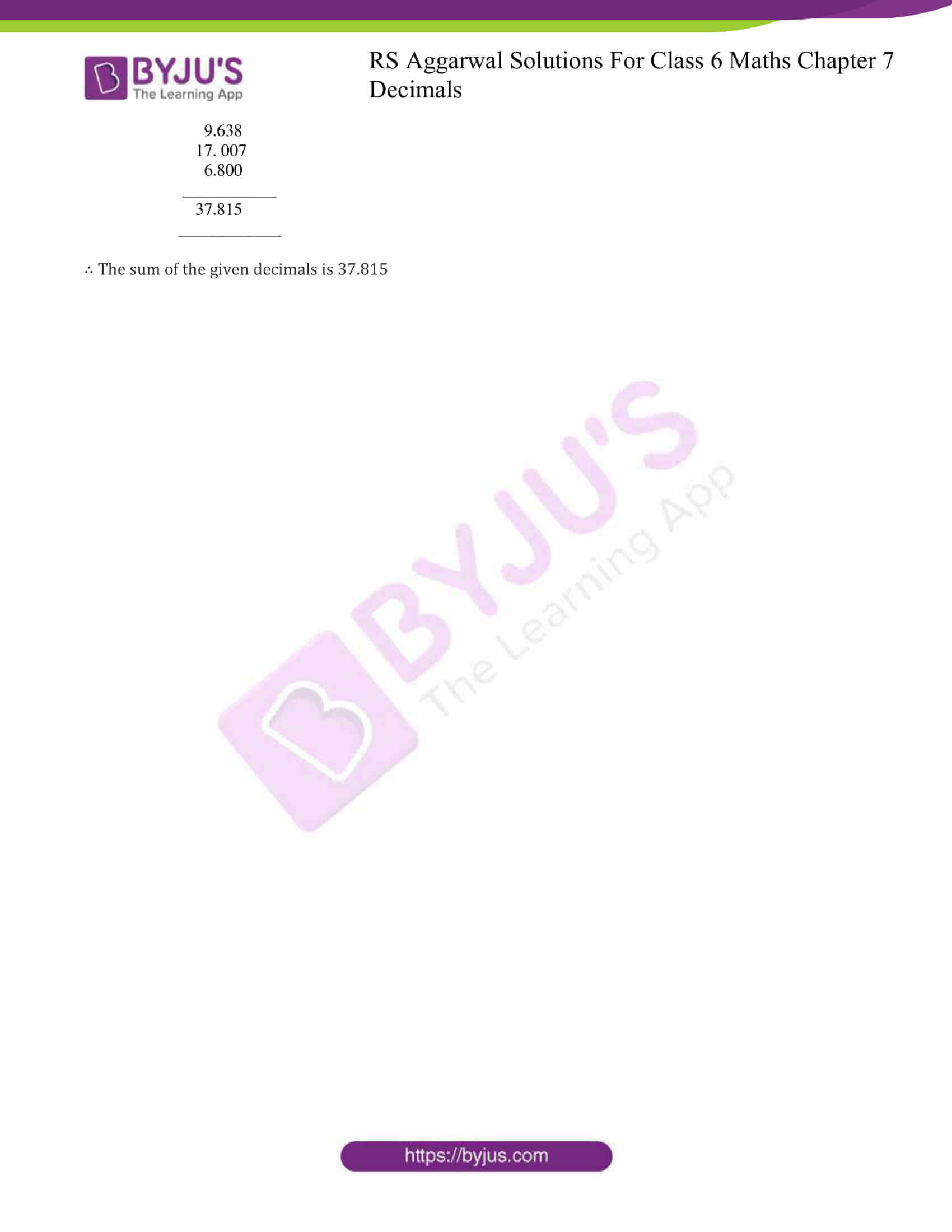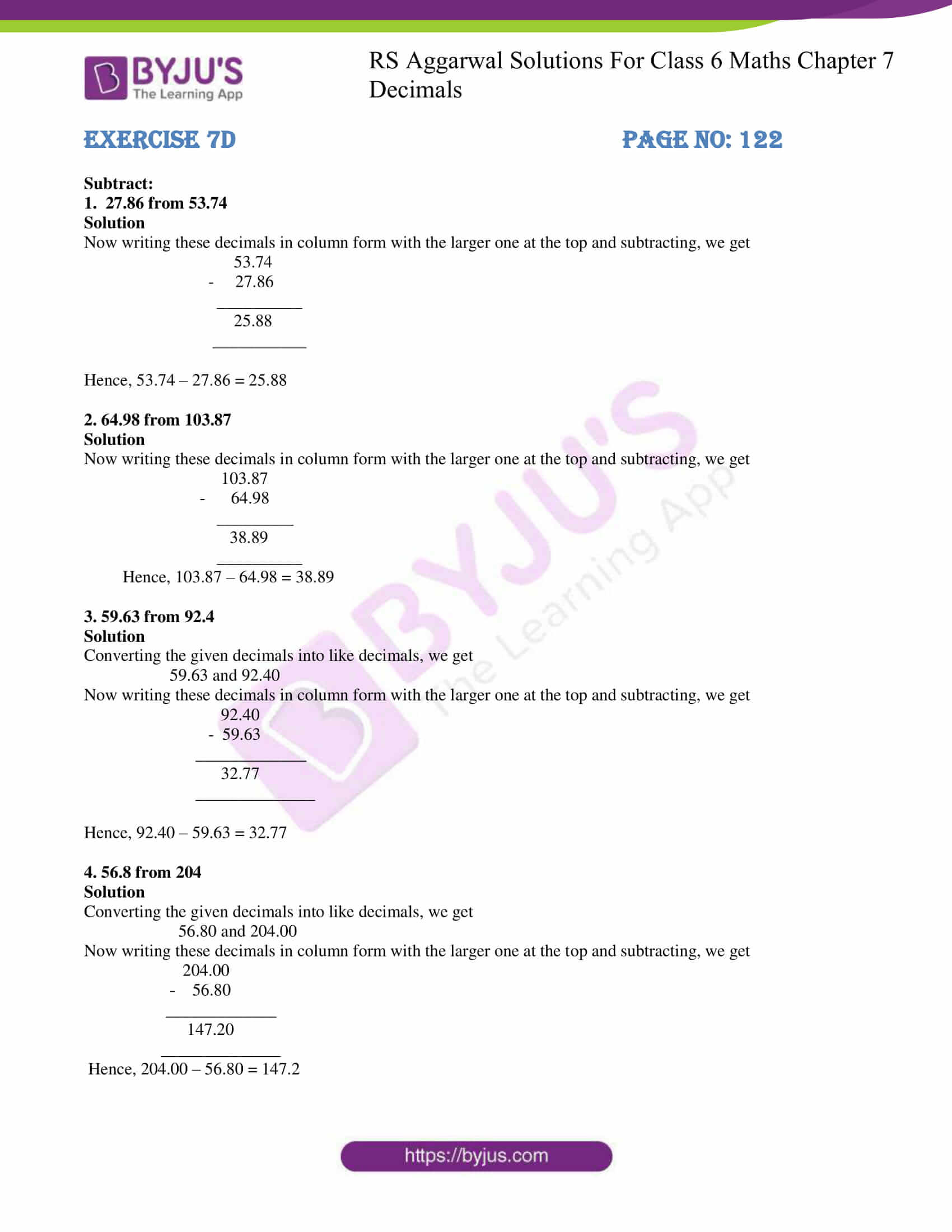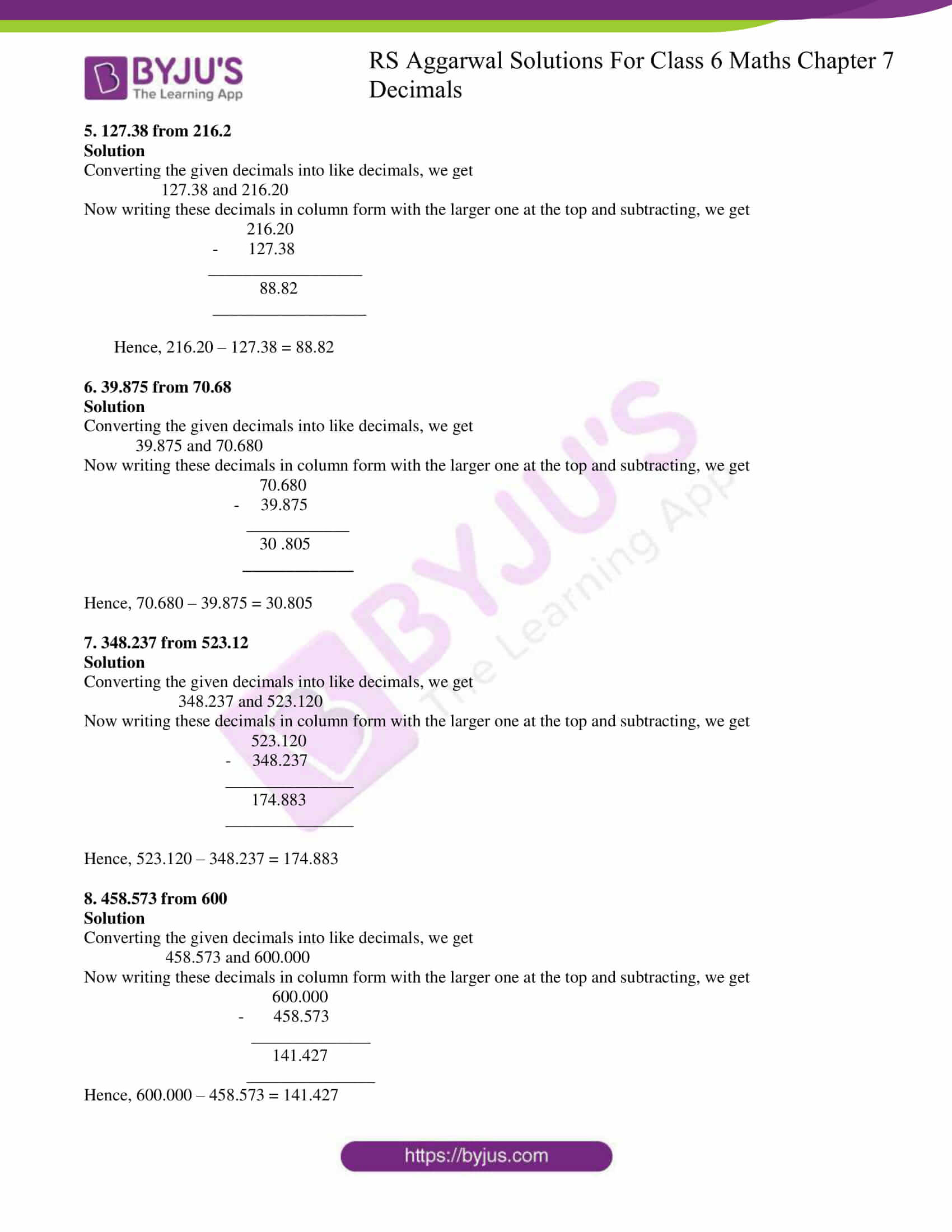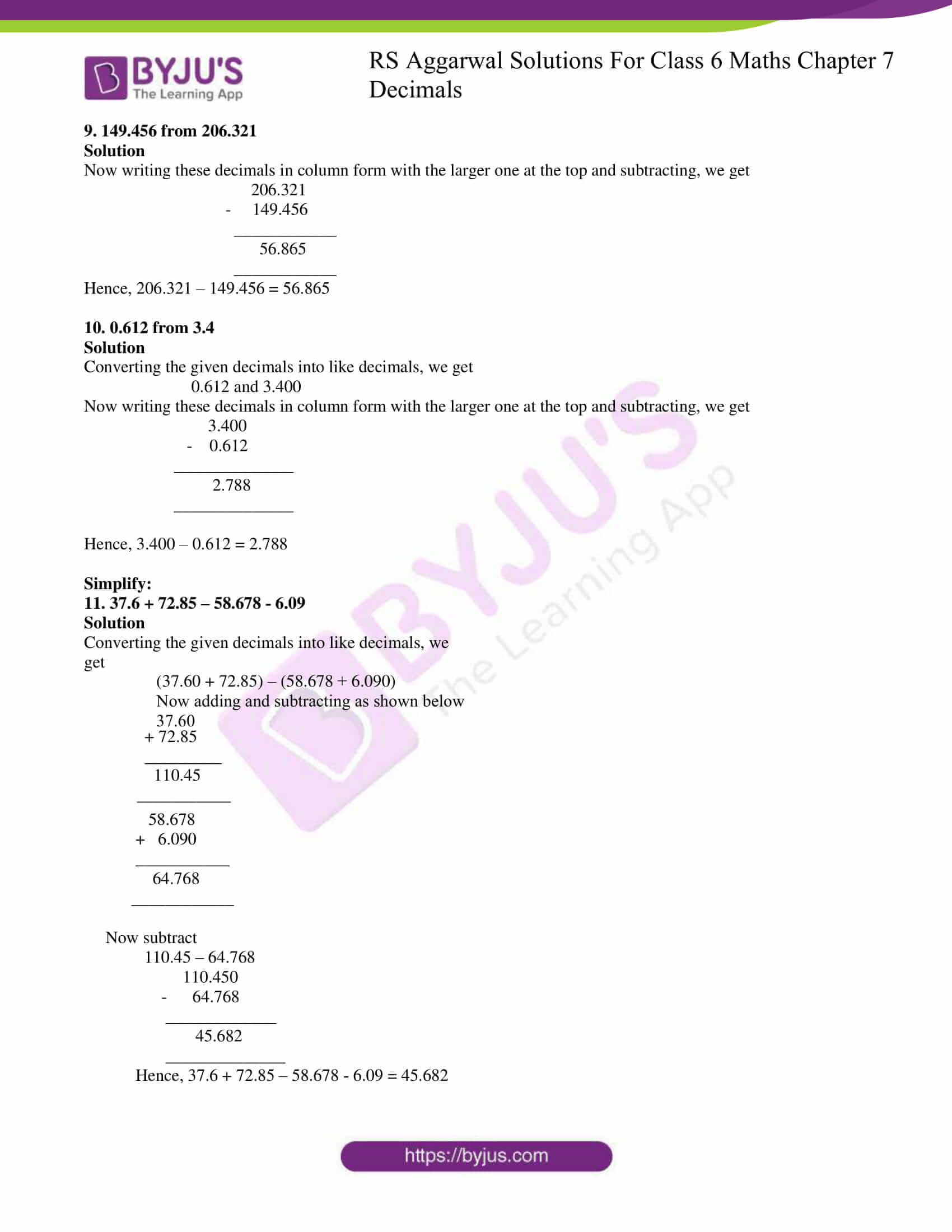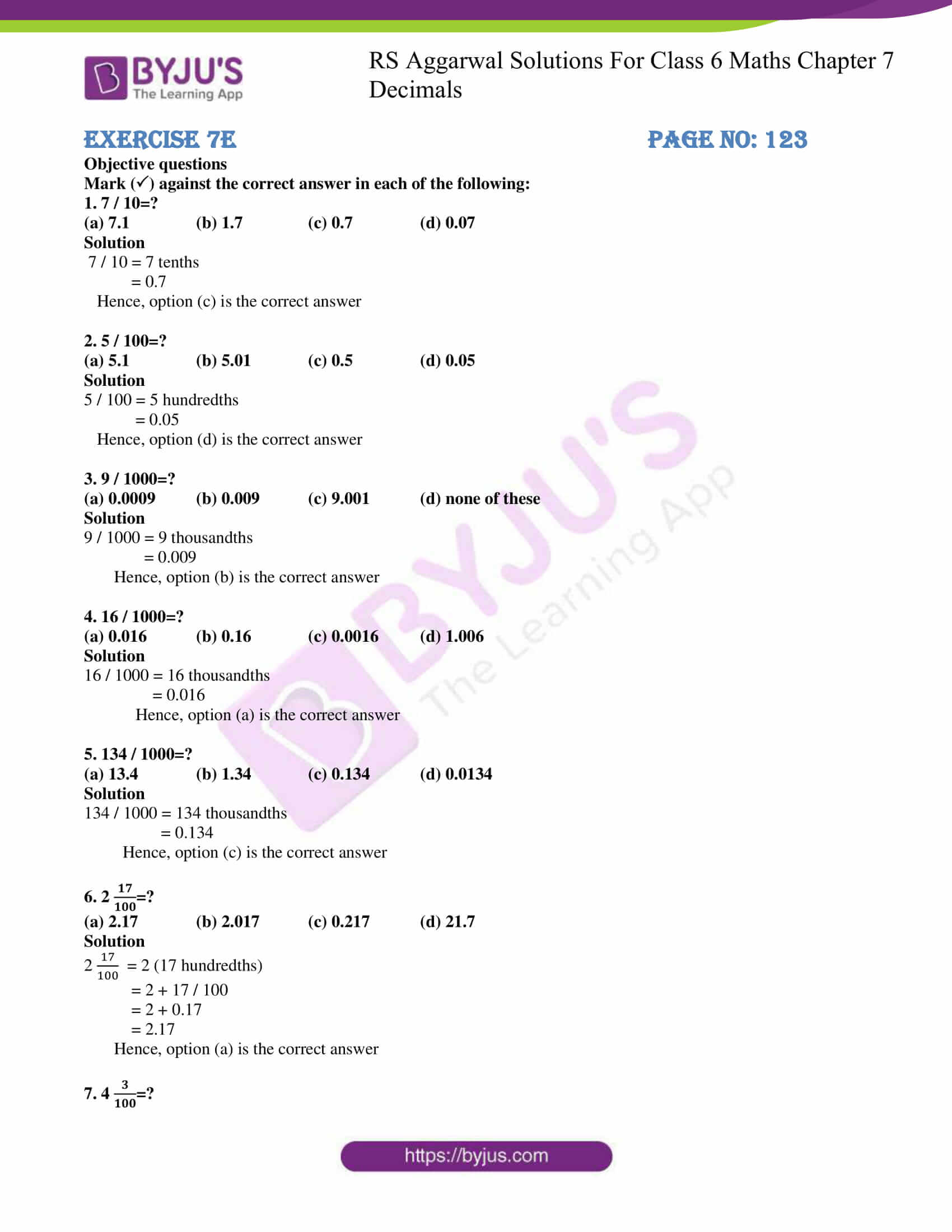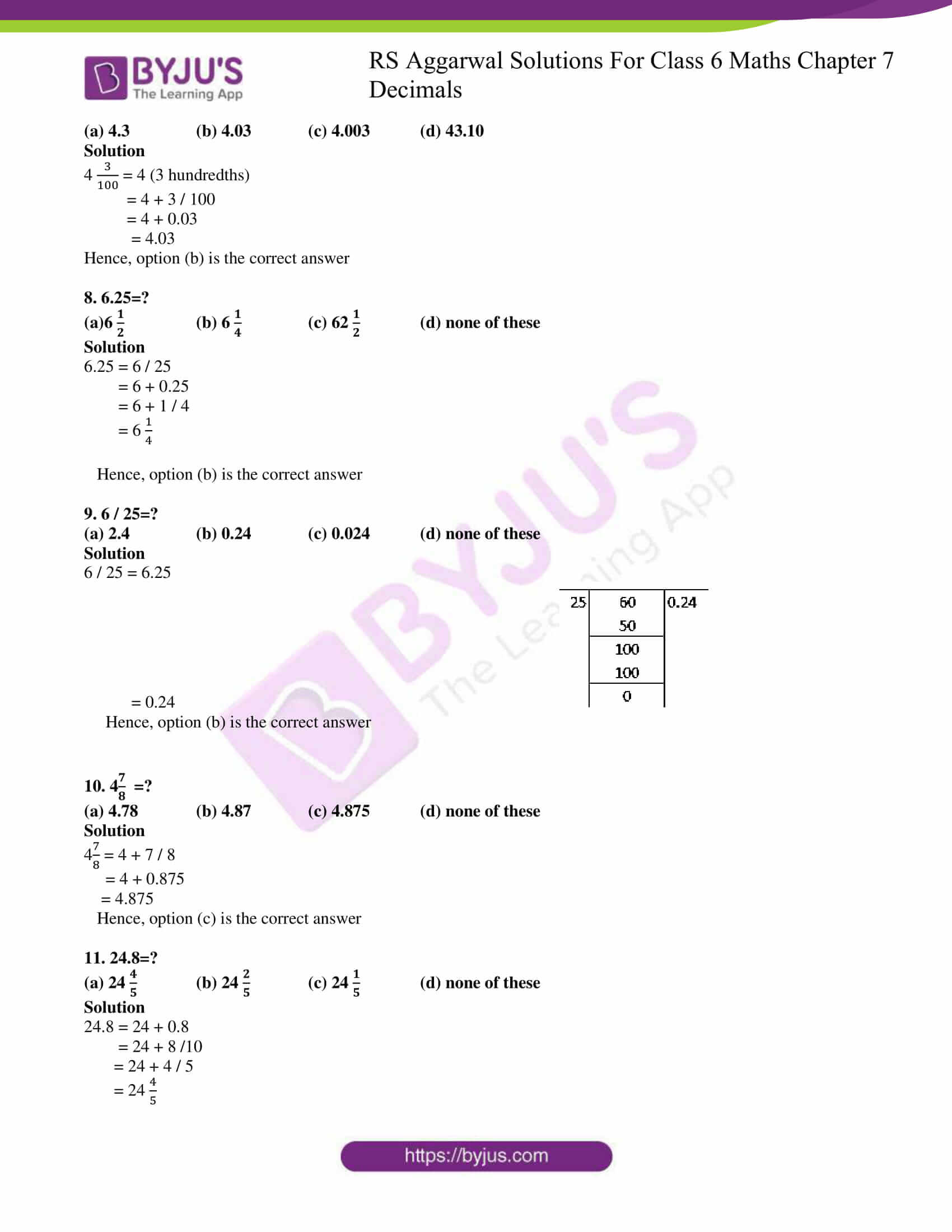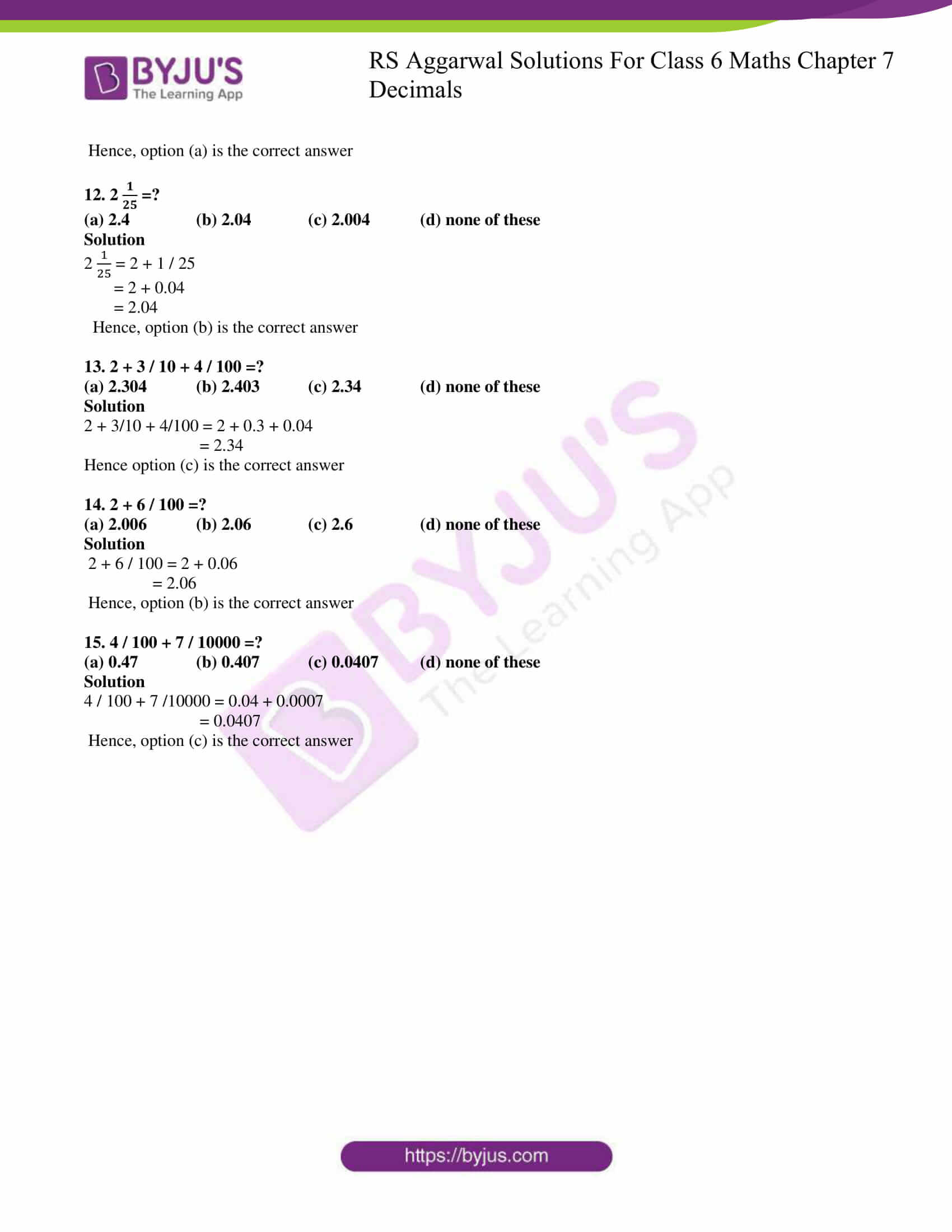## Exercise 7A PAGE NO: 113

1. Write each of the following in figures:

(i) Fifty- eight point six three

(ii) One hundred twenty- four point four two five

(iii) Seven point seven six

(iv) Nineteen point eight

(v) Four hundred four point zero four four

(vi) Point one seven three

(vii) Point zero one five

Solutions

(i) Fifty- eight point six three = 58.63

(ii) One hundred twenty- four point four two five = 124.425

(iii) Seven point seven six = 7.76

(iv) Nineteen point eight = 19.8

(v) Four hundred four point zero four four = 404.044

(vi) Point one seven three = 0 .173

(vii) Point zero one five = 0.015

2. Write the place value of each digit in each of the following decimals:

(i) 14.83

(ii) 275.269

(iii) 46.075

(iv) 302.459

(v) 5370.34

(vi) 186.209

Solutions

(i) 14.83

Here, in 14.83

Place value of 1 = 1 tens

= 10

Place value of 4 = 4 ones

= 4

Place value of 8 = 8 tenths

= 8 / 10

Place value of 3 = 3 hundredths

= 3 / 100

(ii) 275.269

Here, in 275.269

Place value of 2 = 2 hundreds

= 200

Place value of 7 = 7 tens

= 70

Place value of 5 = 5 ones

= 5

Place value of 2 = 2 tenths

= 2 / 10

Place value of 6 = 6 hundredths

= 6 / 100

Place value of 9 = 9 thousandths

= 9 / 1000

(iii) 46.075

Here, in 46.075

Place value of 4 = 4 tens

= 40

Place value of 6 = 6 ones

= 6

Place value of 0 = 0 tenths

= 0 / 10

= 0

Place value of 7 = 7 hundredths = 7 / 100

Place value of 5 = 5 thousandths = 5 / 1000

(iv) 302.459

Here, in 302.459

Place value of 3 = 3 hundreds

= 300

Place value of 0 = 0 tens

= 0

Place value of 2 = 2 ones

= 2

Place value of 4 = 4 tenths

= 4 / 10

Place value of 5 = 5 hundredths

= 5 / 100

Place value of 9 = 9 thousandths

= 9 / 1000

(v) 5370.34

Here, in 5370.34

Place value of 5 = 5 thousands

= 5000

Place value of 3 = 3 hundreds

= 300

Place value of 7 = 7 tens

= 70

Place value of 0 = 0 ones

= 0

Place value of 3 = 3 tenths

= 3 / 10

Place value of 4 = 4 hundredths

= 4 / 100

(vi) 186.209

Here, in 186.209

Place value of 1 = 1 hundreds

= 100

Place value of 8 = 8 tens

= 80

Place value of 6 = 6 ones

= 6

Place value of 2 = 2 tenths

= 2 / 10

Place value of 0 = 0 hundredths

= 0

Place value of 9 = 9 thousandths

= 9 / 1000

3. Write each of the following decimals in expanded form:

(i) 67.83

(ii) 283.61

(iii) 24.675

(iv) 0.294

(v) 8.006

(vi) 4615.72

Solutions

(i) 67.83

= 6 tens + 7 ones + 8 tenths + 3 hundredths

= (6 × 10) + (7 × 1) + (8 × 1 / 10) + (3 × 1 / 100)

= 60 + 7 + 8 / 10 + 3 / 100

∴ 67.83 = 60 + 7 + 8 / 10 + 3 / 100

(ii) 283.61

= 2 hundreds +8 tens + 3 ones + 6 tenths + 1 hundredths

= (2 × 100) + (8 × 10) + (3 × 1) + (6 × 1 / 10) + (1 × 1 / 100)

= 200 + 80 + 3 + 6 / 10 + 1 / 100

∴ 283.61 = 200 + 80 + 3 + 6 / 10 + 1 / 100

(iii) 24.675

= 2 tens + 4 ones + 6 tenths + 7 hundredths + 5 thousandths

= (2 × 10) + (4 × 1) + (6 × 1 / 10) + (7 × 1 / 100) + (5 × 1 / 1000)

= 20 + 4 + 6 / 10 + 7 / 100 + 5 / 1000

∴ 24.675 = 20 + 4 + 6 / 10 + 7 / 100 + 5 / 1000

(iv) 0.294

= 2 tenths + 9 hundredths + 4 thousandths

= (2 × 1 / 10) + (9 × 1 / 100) + (4 × 1 / 1000)

= 2 / 10 + 9 / 100 + 4 / 1000

∴0.294 = 2 / 10 + 9 / 100 + 4 / 1000

(v) 8.006

= 8 ones + 0 tenths + 0 hundredths + 6 thousandths

= (8 × 1) + (0 × 1 /1 0) + (0 × 1 / 100) + (6 × 1 / 1000)

= 8 + 0 / 10 + 0 / 100 + 6 / 1000

= 8 + 6 / 1000

∴8.006 = 8 + 6 / 1000

(vi) 4615.72

= 4 thousands + 6 hundreds + 1 tens + 5 ones + 7 tenths + 2 hundredths

= (4 × 1000) + (6 × 100) + (1 × 10) + (5 × 1) + (7 × 1 / 10) + (2 × 1 / 100)

= 4000 + 600 + 10 + 5 + 7 / 10 + 2 / 100

∴ 4615.72 = 4000 + 600 + 10 + 5 + 7 / 10 + 2 / 100

4. Write each of the following in decimal form:

(i) 40 + 6 + 7 / 10 + 9 / 100

(ii) 500 + 70 + 8 + 3 / 10 + 1 / 100 + 6 / 1000

(iii) 700 + 30 + 1 + 8 / 10 + 4 / 100

(iv) 600 + 5 + 7 / 100 + 9 / 1000

(v) 800 + 5 + 8 / 10 + 6 / 1000

(vi) 30 + 9 + 4 / 100 + 8 / 1000

Solutions

(i) The decimal form of 40 + 6 + 7 / 10 + 9 / 100 = 40 + 6 + 0.7 + 0.09

= 46 + 0.7 + 0.09

= 46.79

(ii) The decimal form of 500 + 70 + 8 + 3 / 10 + 1 / 100 + 6 / 1000

= 500 + 70 + 8 + 0.3 + 0.01 + 0.006

= 578.316

(iii) The decimal form of 700 + 30 + 1 + 8 / 10 + 4 / 100 = 700 + 30 + 1 + 0.8 + 0.04

= 731.84

(iv) The decimal form of 600 + 5 + 7 / 100 + 9 / 1000 = 605 + 0.07 + 0.009

= 605.079

(v) The decimal form of 800 + 5 + 8 / 10 + 6 / 1000 = 805 + 0.8 + 0.006

= 805.806

(vi) The decimal form 30 + 9 + 4 / 100 + 8 / 1000 = 39 + 0.04 + 0.008

= 39.048

## Exercise 7B PaGE nO: 118

Convert each of the following into a fraction in its simplest form:

1. .9

Solution

.9 = 9 / 10

∴ The simplest form of .9 = 9 / 10

2. 0.6

Solution

0.6 = 6 / 10

= 3 / 5

∴ The simplest form of 0.6 = 3 / 5

3. .08

Solution

.08 = 8 / 100

= 4 / 50

= 2 / 25

∴ The simplest form of .08 = 2 / 25

4. 0.15

Solution

0.15 = 15 / 100

= 3 / 20

∴ The simplest form of 0.15 = 3 / 20

5. 0.48

Solution

0.48 = 48 / 100

= 12 / 25

∴ The simplest form of 0.48 = 12 / 25

6. .053

Solution

.053 = 53 / 1000

∴ The simplest form of .053 = 53 / 1000

7. 0.125

Solution

0.125 = 125 / 1000

= 25 / 200

= 5 / 40

= 1 / 8

∴ The simplest form of 0.125 = 1 / 8

8. .224

Solution

.224 = 224 / 1000

= 56 / 250

= 28 / 125

∴ The simplest form of .224 = 28 / 125

Convert each of the following as a mixed fraction:

9. 6.4

Solution

Given

6.4 = 64 / 10

= 32 / 5

= 6 2/5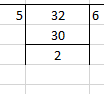∴ Mixed fraction of 6.4 = 6 2 / 5

10. 16.5

Solution

Given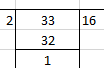16.5 = 165 / 10

= 33 / 2

= 16 1 / 2

∴ Mixed fraction of 16.5 = 16 1 / 2

11. 8.36

Solution

Given

8.36 = 836 / 100

= 209 / 25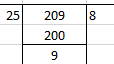= 8 9 / 25

∴ Mixed fraction of 8.36 = 8 9 / 25

12. 4.275

Solution

Given

4.275

= 4275 / 1000

= 171 / 40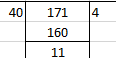= 4 11 / 40

∴ Mixed fraction of 4.275 = 4 11 / 40

13. 25.06

Solution

Given

25.06

= 2506 / 100

= 1253 / 50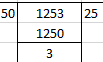= 25 3 / 50

∴ Mixed fraction of 25.06 = 25 3 / 50

14. 7.004

Solution

Given

7.004

= 7004 / 1000

= 1751 / 250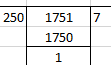= 7 1 / 250

∴ Mixed fraction of 7.004 = 7 1 / 250

15. 2.052

Solution

Given

2.052

= 2052 / 1000

= 513 / 250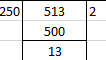= 2 13 / 250

∴ Mixed fraction of 2.052 = 2 13 / 250

16. 3.108

Solution

Given

3.108

= 3108 / 1000

= 777 / 250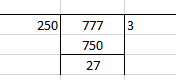= 3 27 / 250

∴ Mixed fraction of 3.108 = 3 27 / 250

Convert each of the following into a decimal:

17. 23 / 10

Solution

Given

23 / 10

By Actual division, we get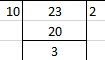= 2 3 / 10

= 2 + 0.3

= 2.3

∴ Decimal form of 23 / 10 = 2.3

18. 167 / 100

Solution

Given

167 / 100

By Actual division, we get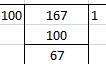= 1 67 / 100

= 1 + 0.67

= 1.67

∴ Decimal form of 167 / 100 = 1.67

## Exercise 7C PAGE NO: 120

1. 9.6, 14.8, 37, and 5.9

Solution

Converting the given decimals into like decimals, we get

9.6, 14.8, 37.0 and 5.9

Writing these decimals in column form and adding, we get

9.6

14.8

37.0

5.9

____________

67.3

_____________

∴ The sum of the given decimals is 67.3

2. 23.7, 106.94, 68.9 and 29.5

Solution

Converting the given decimals into like decimals, we get

23.70, 106.94, 68.90 and 29.50

Now writing these decimals in column form and adding, we get

23.70

106.94

68.90

29.50

______________

229.04

_______________

∴ The sum of the given decimals is 229.04

3. 72.8, 7.68, 16.23 and 0.7

Solution

Converting the given decimals into like decimals, we get

72.80, 7.68, 16.23 and 0.70

Now writing these decimals in column form and adding, we get

72.80

7.68

16.23

0.70

_____________

97.41

______________

∴ The sum of the given decimals is 97.41

4. 18.6, 84.75, 8.345 and 9.7

Solution

Converting the given decimals into like decimals, we get

18.600, 84.750, 8.345 and 9.700

Now writing these decimals in column form and adding, we get

18.600

84.750

8.345

9.700

________________

121.395

_________________

∴ The sum of the given decimals is 121.395

5. 8.236, 16.064, 63.8 and 27.53

Solution

Converting the given decimals into like decimals, we get

8.236, 16.064, 63.800 and 27.530

Now writing these decimals in column form and adding, we get

8.236

16.064

63.800

27.530

___________

115.630

___________

∴ The sum of the given decimals is 115.63

6. 28.9, 19.64, 123.697 and 0.354

Solution

Converting the given decimals into like decimals, we get

28.900, 19.640, 123.697 and 0.354

Now writing these decimals in column form, we get

28.900

19.640

123.697

0.354

___________

172.591

____________

∴ The sum of the given decimals is 172.591

7. 4.37, 9.638, 17.007 and 6.8

Solution

Converting these decimals into like decimals, we get

4.370, 9.638, 17.007 and 6.800

Now writing these decimals in column form, we get

4.370

9.638

17. 007

6.800

___________

37.815

____________

∴ The sum of the given decimals is 37.815

## Exercise 7D PAGE no: 122

Subtract:

1. 27.86 from 53.74

Solution

Now writing these decimals in column form with the larger one at the top and subtracting, we get

53.74

– 27.86

__________

25.88

___________

Hence, 53.74 – 27.86 = 25.88

2. 64.98 from 103.87

Solution

Now writing these decimals in column form with the larger one at the top and subtracting, we get

103.87

– 64.98

_________

38.89

__________

Hence, 103.87 – 64.98 = 38.89

3. 59.63 from 92.4

Solution

Converting the given decimals into like decimals, we get

59.63 and 92.40

Now writing these decimals in column form with the larger one at the top and subtracting, we get

92.40

– 59.63

_____________

32.77

______________

Hence, 92.40 – 59.63 = 32.77

4. 56.8 from 204

Solution

Converting the given decimals into like decimals, we get

56.80 and 204.00

Now writing these decimals in column form with the larger one at the top and subtracting, we get

204.00

– 56.80

_____________

147.20

______________

Hence, 204.00 – 56.80 = 147.2

5. 127.38 from 216.2

Solution

Converting the given decimals into like decimals, we get

127.38 and 216.20

Now writing these decimals in column form with the larger one at the top and subtracting, we get

216.20

– 127.38

__________________

88.82

__________________

Hence, 216.20 – 127.38 = 88.82

6. 39.875 from 70.68

Solution

Converting the given decimals into like decimals, we get

39.875 and 70.680

Now writing these decimals in column form with the larger one at the top and subtracting, we get

70.680

– 39.875

____________

30 .805

_____________

Hence, 70.680 – 39.875 = 30.805

7. 348.237 from 523.12

Solution

Converting the given decimals into like decimals, we get

348.237 and 523.120

Now writing these decimals in column form with the larger one at the top and subtracting, we get

523.120

– 348.237

_______________

174.883

_______________

Hence, 523.120 – 348.237 = 174.883

8. 458.573 from 600

Solution

Converting the given decimals into like decimals, we get

458.573 and 600.000

Now writing these decimals in column form with the larger one at the top and subtracting, we get

600.000

– 458.573

______________

141.427

_______________

Hence, 600.000 – 458.573 = 141.427

9. 149.456 from 206.321

Solution

Now writing these decimals in column form with the larger one at the top and subtracting, we get

206.321

– 149.456

____________

56.865

____________

Hence, 206.321 – 149.456 = 56.865

10. 0.612 from 3.4

Solution

Converting the given decimals into like decimals, we get

0.612 and 3.400

Now writing these decimals in column form with the larger one at the top and subtracting, we get

3.400

– 0.612

______________

2.788

______________

Hence, 3.400 – 0.612 = 2.788

Simplify:

11. 37.6 + 72.85 – 58.678 – 6.09

Solution

Converting the given decimals into like decimals, we get

(37.60 + 72.85) – (58.678 + 6.090)

Now adding and subtracting as shown below

37.60

+ 72.85

_________

110.45

___________

58.678

+ 6.090

___________

64.768

____________

Now subtract

110.45 – 64.768

110.450

– 64.768

_____________

45.682

______________

Hence, 37.6 + 72.85 – 58.678 – 6.09 = 45.682

## Exercise 7E PAGE NO: 123

Objective questions

Mark () against the correct answer in each of the following:

1. 7 / 10=?

(a) 7.1 (b) 1.7 (c) 0.7 (d) 0.07

Solution

7 / 10 = 7 tenths

= 0.7

Hence, option (c) is the correct answer

2. 5 / 100=?

(a) 5.1 (b) 5.01 (c) 0.5 (d) 0.05

Solution

5 / 100 = 5 hundredths

= 0.05

Hence, option (d) is the correct answer

3. 9 / 1000=?

(a) 0.0009 (b) 0.009 (c) 9.001 (d) none of these

Solution

9 / 1000 = 9 thousandths

= 0.009

Hence, option (b) is the correct answer

4. 16 / 1000=?

(a) 0.016 (b) 0.16 (c) 0.0016 (d) 1.006

Solution

16 / 1000 = 16 thousandths

= 0.016

Hence, option (a) is the correct answer

5. 134 / 1000=?

(a) 13.4 (b) 1.34 (c) 0.134 (d) 0.0134

Solution

134 / 1000 = 134 thousandths

= 0.134

Hence, option (c) is the correct answer

6. 2 17/100 =?

(a) 2.17 (b) 2.017 (c) 0.217 (d) 21.7

Solution

2 = 2 (17 hundredths)

= 2 + 17 / 100

= 2 + 0.17

= 2.17

Hence, option (a) is the correct answer

7. 4 3/100 =?

(a) 4.3 (b) 4.03 (c) 4.003 (d) 43.10

Solution

4 = 4 (3 hundredths)

= 4 + 3 / 100

= 4 + 0.03

= 4.03

Hence, option (b) is the correct answer

8. 6.25=?

(a)6 1/2 (b) 6 1/4 (c) 62 1/2 (d) none of these

Solution

6.25 = 6 / 25

= 6 + 0.25

= 6 + 1 / 4

= 6 1/4

Hence, option (b) is the correct answer

9. 6 / 25=?

(a) 2.4 (b) 0.24 (c) 0.024 (d) none of these

Solution

6 / 25 = 6.25

= 0.24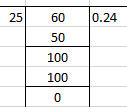Hence, option (b) is the correct answer

10. 4 7/8 =?

(a) 4.78 (b) 4.87 (c) 4.875 (d) none of these

Solution

4 = 4 + 7 / 8

= 4 + 0.875

= 4.875

Hence, option (c) is the correct answer

11. 24.8=?

(a) 24 4/5 (b) 24 2/5 (c) 24 1/5 (d) none of these

Solution

24.8 = 24 + 0.8

= 24 + 8 /10

= 24 + 4 / 5

= 24 4/5

Hence, option (a) is the correct answer

12. 2 1/25 =?

(a) 2.4 (b) 2.04 (c) 2.004 (d) none of these

Solution

2 = 2 + 1 / 25

= 2 + 0.04

= 2.04

Hence, option (b) is the correct answer

13. 2 + 3 / 10 + 4 / 100 =?

(a) 2.304 (b) 2.403 (c) 2.34 (d) none of these

Solution

2 + 3/10 + 4/100 = 2 + 0.3 + 0.04

= 2.34

Hence option (c) is the correct answer

14. 2 + 6 / 100 =?

(a) 2.006 (b) 2.06 (c) 2.6 (d) none of these

Solution

2 + 6 / 100 = 2 + 0.06

= 2.06

Hence, option (b) is the correct answer

15. 4 / 100 + 7 / 10000 =?

(a) 0.47 (b) 0.407 (c) 0.0407 (d) none of these

Solution

4 / 100 + 7 /10000 = 0.04 + 0.0007

= 0.0407

Hence, option (c) is the correct answer

### RS Aggarwal Solutions for Class 6 Maths Chapter 7 Decimals

Chapter 7 – Decimals consists of 5 exercises. For each question, RS Aggarwal Solutions are solved in detail in every exercise. Let’s have a look at the topics mentioned in this chapter

• Decimals
• Like and Unlike decimals
• Converting a decimal into a fraction
• Converting a fraction into a decimal
• Operations on decimals

### Chapter Brief of RS Aggarwal Solutions for Class 6 Maths Chapter 7 Decimals

A number expressed in the scale of tens is known as a decimal. Decimals are fractions in which the denominators are 10, 100, 1000, etc. Decimals having the same number of decimal places are called like decimals and those having a different number of decimal places are called unlike decimals. These are used while dealing with money, weight and length.